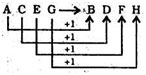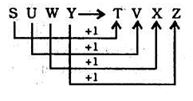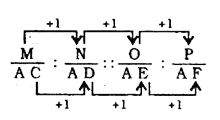# JP-Morgan Reasoning Question

DIRECTIONS for questions 1 – 2: In each of the following questions, select the related letters/word/number from the given alternatives.
Q, U, X, D, A, B and N are sitting around a circle facing at the center. D is third to the left of X who is second to the left of Q. U is third to the left of D and second to the right of A. B is not an immediate neighbor of Q.
1. ACEG : SUWY : : BDFH : ?
1. TVZX
2. RTZV
3. TVXZ
4. RTVZSimilarly,1. M/AC : N/AD = O/AC : ?
1. P / AF
2. Q / AB
3. P / AC2. If the day before yesterday was Sunday, what day will it be three days after the day after tomorrow?
1. Sunday
2. Monday
3. Wednesday
4. Saturday
Day before yesterday was Sunday.
Therefore, today is Tuesday. Day after tomorrow will be Thurs¬day. Thursday + 3 = Sunday
3. What is the number missing from the third target?1. 45
2. 48
3. 51
4. 54
5 × 3 + 1 = 16
16 × 3 + 1 = 49
9 × 3 + 2 = 29
29 × 3 + 2 = 89
Therefore,
15 × 3 + 3 = 
48 × 3 + 3 = 147
4. In a classroom, there are 5 rows, and 5 children A, B, C. D and E are seated one behind the other in 5 separate rows as follows:
A is sitting behind C, but in front of B.
C is sitting behind E. D is sitting in front of E.
The order in which they are sitting from the first row to the last is:
1. DECAB
2. BACED
3. ACBDE
4. ABEDC
1st Row ⇒ D
2nd Row ⇒ E
3rd Row ⇒ C
4th Row ⇒ A
5th Row ⇒ B
5. Find out a set of numbers amongst the four sets of numbers given in the alternatives, which is the most similar to the numbers given in the' question. Given : (6, 30, 90)
1. 6, 42, 86
2. 7, 42, 218
3. 6, 24, 70
4. 8, 48, 192
6 × 5 = 30,
30 × 3 = 90
8 × 6 = 48,
48 × 4 = 192
6. In a row of boys, Srinath is 7th from the left and Venkat is 12th from the right. If they interchange their positions, Srinath becomes 22nd from the left. How many boys are there in the row?
1. 19
2. 31
3. 33
4. 34Total number of boys in the row = 22 +12 - 1 = 33
DIRECTIONS for questions 8 – 9: In each of the following questions, select the one which is different from the other three responses.
1. Wave
2. Current
3. Tide
4. Storm
Except .Tide, all other terms are related to both air and water. But tide is a regular rise and fall in the level of sea, caused by the attraction of the moon and sun.
1. X
2. Y
3. H
4. D
The position number of Y in the English alphabet is an odd number.
X ⇒ 24, Y ⇒ 25,
H ⇒ 8, D ⇒ 4
7. Insert the correct missing number from the choices given below
 2 cm 7 cm 9 cm 18 cm 36 cm 72 cm 157 150 141 123 87 ?
1. 36
2. 15
3. 51
4. 69